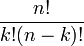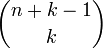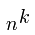## 17 May 2011

### The Combinatorial and Other Math of the Java Collections API

The Java Collections API both involves some explicit Math and presents some nice artifacts which parallel other Math concepts.

Combinatorics is probably one of the more fundamental Maths for Computer Science and is also prevalent throughout other Maths. In one example of Combinatorial counting problems, there is a collection of items, say a deck of cards, from which we want to determine the number of selections, say hands. The formula to compute the possible Combinations is written as:

This can also be stated as “n choose k.” My mnemonic for remembering this, and it is worth remembering, is to look at the left side above note that the n is on top and the k is on the bottom, which visually maps the n! to the numerator and k! to the denominator, and then rotate the left part by 90 degrees counter clockwise so that it forms (n - k)! and put it in the denominator. Also every number calculated by this equation is a Binomial Coefficient found in Pascal’s Triangle, which in my opinion is one of the most amazing mathematical structures.

Our example for possible five card hands from a deck of cards computes as follows:

Combinations give you the number of elements with no repetitions and where order does not matter. The possible cases and Mathematical structures for the criteria of order and repetition are as follows:

 Order Does not Matter Order Matters No Repetitions Combination/Set Permutation Repetitions Multiset/Bag Enumeration/List/String

The Combinatorial equations are as follows:

 Order Does not Matter Order Matters No RepetitionsRepetitionsThe interesting thing about lists is that if you make lists of varying lengths of the following characters [0,1,2,3,4,5,6,7,8,9] , you have the natural numbers in base ten, so a list of length 3 gives you 103 or 1000 possibilities, which are: 000-999. Also lists have the highest cardinality of the four:

|List| > |Multiset| > |Permutation| > |Combinitation|

Where |object| means the cardinality or size of the collection, which is the number of elements in the set, list, etc.

The corresponding Java classes/interfaces are:

 Order Does not Matter Order Matters No Repetitions java.util.Set java.util.SortedSet Repetitions org.apache.commons.collections.Bag java.util.List/java.lang.String

Sadly the Java Collections API is incomplete in this regard. Also this example is somewhat abstract but it does demonstrate how to view these objects from a Combinatorial perspective, which in turn can help with choosing the appropriate data structure, also it gives insight into possible ways that they may be populated and perhaps even tested.

This is an introductory example to Combinatorics, for more perspective there is an expansion on this known as the Twelvefold way.

The next example is more concrete, in fact it comes directly from the java docs for Comparator and Comparable:

For the mathematically inclined, the relation that defines the imposed ordering that a given comparator c imposes on a given set of objects S is:
```{(x, y) such that c.compare(x, y) <= 0}.
```
The quotient for this total order is:
```{(x, y) such that c.compare(x, y) == 0}.
```
It follows immediately from the contract for compare that the quotient is an equivalence relation on S, and that the imposed ordering is a total order on S. When we say that the ordering imposed by c on S is consistent with equals, we mean that the quotient for the ordering is the equivalence relation defined by the objects' `equals(Object)` method(s):
`{(x, y) such that x.equals(y)}. `

I have to admit when I first read that a few years back, I was like, WTF does that mean! Obviously whoever wrote that was thinking deeply and mathematically about the underlying principals here which is clearly needed if you are designing fundamental low level framework aspects of base classes and collections.

Comparators and Comparable are about ordering, so what is being defined above is a Total Order which means that all elements can be compared to every other element, compare this to a Partial Order where elements may or may not be comparable to each other, both of these fall under the more general Order Theory. The Quotient aka Equivalence Class concept is a bit more complicated and beyond what I am trying to talk about here.

I believe the above Comparator Javadoc quote was written by Joshua Bloch, the following is from his book Effective Java:

The equals method implements an equivalence relation. It is:
• Reflexive: For any non-null reference value x, x.equals(x) must return true.
• Symmetric: For any non-null reference values x and y, x.equals(y) must return true if and only if y.equals(x) returns true.
• Transitive: For any non-null reference values x, y, z, if x.equals(y) returns true and y.equals(z) returns true, then x.equals(z) must return true.
• Consistent: For any non-null reference values x and y, multiple invocations of x.equals(y) consistently return true or consistently return false, provided no information used in equals comparisons on the objects is modified.
• For any non-null reference value x, x.equals(null) must return false.

The first three are the Mathematical definition of an Equivalence Relation (which relates back to the Equivalence Class) can be found in “Abstract Mathematics” or “Transition to Advanced Mathematics” type books which describe Functions and Relations. CS Majors usually are exposed to these concepts as part of the intro into discrete math.

What I find interesting about these concepts is how the comparator/comparable and equals relation and their interrelationship for the individual object map back to control the collections behavior of ordering and repetitions.

1.2.